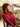# Getting started with EJS

Posted on September 17, 2017 - 4 min read

An understanding of how EJS works

This is a short explanation of how I finally understood and started using EJS in most of the basic applications that I've been working on. First, before moving forward let me explain what EJS is and where exactly you might need to use it for those who don't already know.

Where is EJS used ?: EJS is used in node.js when working with the Express framework as a templating engine to help render JavaScript code on the client-side.

What is EJS ?: EJS stands for embedded JavaScript. It lets us embed JavaScript code (variables, if statement, loops) inside of our HTML. For example

`<h1> Example variable name: exampleVar </h1>`

But the above code won't work yet, because we have to tell HTML that the `exampleVar` is a JavaScript code and it should be treated as one rather than just outputting it as plain HTML and that's where EJS comes in, it's a little bit weird at first when you see it, but this is what a typical EJS looks like: `<%= %>` you have the less than and the percentage sign, space where your JavaScript code goes in, then the percent, followed by the greater than sign.

``````<%= 5 + 5 %>

// this outputs 10``````

but in regular HTML `5 + 5` still gives you `5 + 5`, the same way you typed it. Now going back to our previous example with the variable, we can add whatever variable we are passing from the server-side to the clients' side using EJS and it won't be rendered as HTML. Example of a server-side code:

``````// server-side code

app.get("/", function(req, res) {
res.render("index.ejs", { exampleVar: Javascript })
})

//the "exampleVar" being the name of our variable from the EJS file
//the "JavaScript" the value assigned to the variable``````

Now when we run our previous HTML file with the `.ejs extension` after adding in the EJS brackets our JavaScript code gets rendered correctly.

``````<h1> Example variable name: <%= exampleVar %> </h1>

//"exampleVar" gets outputted as the value "JavaScript"``````

But remember that exampleVar is not HTML, it's a JavaScript variable meaning you could also do things like `<%= exampleVar.toUpperCase() %>` and it would still get rendered correctly.

Besides adding just variable we could also do other things like control flows (if statements, loops, etc)

EJS If Statements: Still using our previous examples, let's add a bit of logic that will check if the value of the variable is `JavaScript`, and also prints out something if it is. So we can do this in the EJS file:

``````<% if( exmapleVar === "JavaScript") { %>
<p> Good Choice </p>
<% } %>

//this will add only the contents of the paragraph to our HTML, if the condition is true``````

Looking at the above example you will notice that every line of JavaScript code is between the EJS brackets, no matter where the code starts or stops it's all wrapped inside the EJS brackets, but if you compare this example with the one above you would notice that we have different forms of the brackets that are this `<%= %>` and this `<% %>` and the difference between these two is that when the `=` is added, whatever is in the space is being returned to the HTML like our `exampleVar` or the `5 + 5` but when doing plain logic like in our if statement or loops we don't want the logic to be added to the HTML so we just wrap it up in the brackets without the `=` sign.

More examples using Loops: Counting the numbers 1 through 10 in plain JavaScript often results in one using a loop

``````for (var i = 1; i < 10; i++) {
console.log(i) //this will output the numbers from 1 - 10
}``````

This can also be done with EJS in a slightly different way, which requires your JavaScript code to be wrapped up in the EJS brackets, so the for loop will then look like this:

``````<% for(var i = 1; i <= 10; i++) { %>
<%= i %>  <%# this will output the numbers from 1 - 10 %>
<%  } %>

// Note: comments in EJS are written between the <%# %> brackets``````

You can also use this for loop example to run through a post array coming from the server-side and sending the contents of each post to the HTML through your EJS file.

I hope this has been somewhat helpful as to get you started with using EJS as your templating engine when working with express on node.js.

As always, Thank you for reading!